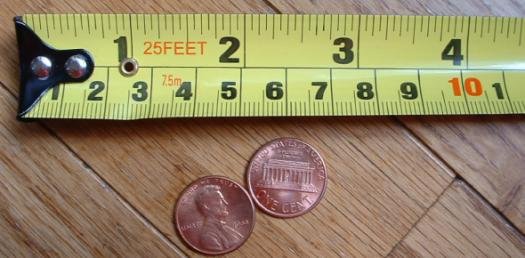# Level Measurement MCQ Quiz With Answers

15 Questions | Total Attempts: 4795SettingsAre you ready for this level measurement quiz that we have here for you? I this quiz, you will find questions on what level is and how continuous level measurement differs from single-point level detection. You need to be well-informed on this subject to score high on this quiz, as it is not going to be that easy challenge for you. So, do you think you are ready for this? Let us see as you take this quiz.

• 1.
"An electrical probe is used to detect a high level in a container. What type of level measurement does the arrangement represent?"
• A.

A. Restrictive level measurement

• B.

B. Continuity level measurement

• C.

C. Single-point level detection

• D.

D. Nutating level detection

• 2.
"Which level measurement devices work on the principle that liquid levels equalize in containers that are connected together?"
• A.

A. Gauge glass

• B.

B. Float and tape

• C.

C. Conductivity probes

• D.

D. Bubbler system

• 3.
"Which type of measurement device is a plumb bob?"
• A.

A. Direct level measurement device

• B.

B. Direct flow measurement device

• C.

C. Indirect level measurement device

• D.

D. Turbulent flow measurement device

• 4.
"The gauge mounted at the bottom of a tank relies on hydrostatic pressure to provide a level indication. What type of level measurement device is it?"
• A.

A. Direct

• B.

B. Conductive

• C.

C. Indirect

• D.

D. Multipoint

• 5.
"Select all of the choices that describe what the level of the indication on the gauge in a bubbler system represents."
• A.

A. The flow rate through the system

• B.

B. The amount of air pressure needed to clear the pipe

• C.

C. The hydrostatic pressure of the liquid above the pipe opening

• D.

D. The temperature of the liquid in the tank

• 6.
"Select all of the statements that are true of the differential pressure cell on a tank."
• A.

A. It can measure the level in a pressurized tank.

• B.

B. It uses a float and tape to measure level.

• C.

C. It measures the difference between two related pressures.

• D.

D. It is a direct-level measurement device.

• 7.
"Which of these terms is defined as the position of the surface of a liquid or a solid above or below a fixed reference point?"
• A.

A. Pressure

• B.

B. Level

• C.

C. Volume

• D.

D. Zero point

• 8.
"Level measurement instruments may be designed for either continuous level measurement or what else?"
• A.

A. Restrictive level measurement

• B.

B. Continuity level measurement

• C.

C. Single-point level detection

• D.

D. Nutating level detection

• 9.
"Select the statement that best describes the operating principle on which the use of a gauge glass is based."
• A.

A. A gauge glass is controlled by the flow of pressurized air.

• B.

B. The level in a gauge glass is half that in a tank.

• C.

C. Liquid levels equalize in containers that are connected together.

• D.

D. Fluid flow in a gauge glass varies with the size of the orifice plate in the tank.

• 10.
"Which of these direct level measurement devices has a weight attached to the end of a reel of a flexible measuring tape?"
• A.

A. Float and cable

• B.

B. Conductivity probe

• C.

C. Resistance tape

• D.

D. Plumb bob

• 11.
"Which physical factor does an indirect level measurement device use to provide a level indication?"
• A.

A. Hydrostatic pressure

• B.

B. Conductivity

• C.

C. Fluid volume

• D.

D. Turbulence

• 12.
"The pipe in a bubbler system is open at the bottom but connected at the top to a pressure-sensitive gauge, and what else?"
• A.

A. A similar tank

• B.

B. A suction device

• C.

C. A regulated air supply

• D.

D. An orifice plate

• 13.
"A D/P cell is connected by tubing to a pressurized tank containing a liquid. Which choice best describes the pressure that's exerted through the tubing that runs from the bottom of the tank to the D/P cell?"
• A.

A. Only the hydrostatic pressure of the liquid

• B.

B. Only the pressure added to the tank.

• C.

C. Atmospheric pressure

• D.

D. Hydrostatic pressure and the pressure added to the tank

• 14.
1. "If the zero point is at the top of a container, how would the level of a material in the container be measured?"
• A.

A. From the bottom of the container up to the surface

• B.

B. From the bottom of the container up to the top, then back down to the surface.

• C.

C. From the top of the container down to the surface

• D.

D. From the bottom of the container to the zero point

• 15.
"The gauge on a tank provides an indication of the level at all times. What category of level measurement would you say it represents?"
• A.

A. Resistive

• B.

B. Multipoint

• C.

C. Continuous

• D.

D. Conductive

Related TopicsBack to top